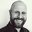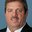Question-and-Answer Resource for the Building Energy Modeling Community
Get started with the Help page

# Correction in OpenStudio - Coil:Cooling:DX:SingleSpeedI think there is a mistake in the graphical interface OpenStudio when configuring the component Coil:Cooling:DX:SingleSpeed.

In the field Rated Evaporator Fan Power per Volume Flow Rate (see figure below), the unit is being shown in Pa. According to the EnergyPlus Input Output Reference it should be in W/(m³/s).Perhaps this relationship translates into Pa, but I do not think so.

edit retag close merge delete

Sort by » oldest newest most votedYou are correct. The E+ idd shows, and code confirms, that the units are W/(m3/s). This input is a normalized power per flow rate input for the fan power used during the rating calculations. This input is not used otherwise.

Coil:Cooling:DX:SingleSpeed,
N5 , \field Rated Evaporator Fan Power Per Volume Flow Rate
\note Enter the evaporator fan power per air volume flow rate at the rated test conditions.
\note The test conditions vary external static pressure based on total cooling capacity.
\note This value is only used to calculate Seasonal Energy Efficiency Ratio (SEER), Energy
\note Efficiency Ratio (EER), Integrated Energy Efficiency Ratio (IEER), and the Standard
\note Rating (Net) Cooling Capacity which will be outputs in the EnergyPlus eio file.  This
\note value is not used for modeling the evaporator fan during simulations.
\type real
\units W/(m3/s)
\minimum 0.0
\maximum 1250.0
\default 773.3

more

I didn't even think about it until Blake showed me the answer.

Another way to look at this is using the fan power equation.

Pfan = (Volume Flow x Pressure)/Fan Efficiency

or in units:

Watts = (m3/s * Pa) / dimensionless number, so W/m3/s = Pa.

@Pithon did you ever create a GitHub issue on this. I think we can and should prevent OS from "reducing" the units like that.That unit breaks down into Pascals, although reading it as W/(m^3/s) is more intuitive.

W/(m^3/s) = (J/s)/(m^3/s) = J/m^3 = N*m/m^3 = N/m^2 = Pa

more